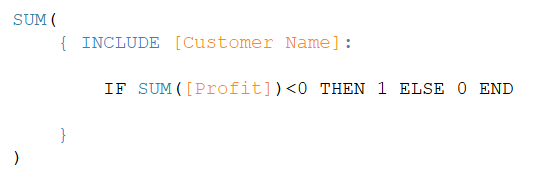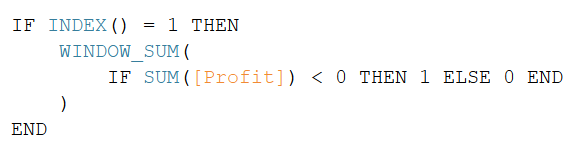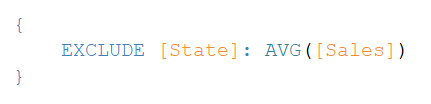The introduction of Level of Detail (LOD) Expressions in Tableau 9.0 was a breakthrough in the area of Calculations. LOD Expressions simplify and extend Tableau’s calculation language to address level-of-detail questions directly. There are some business questions which can only be solved using the LOD Expressions and that’s where they stand out; however, there are certain scenarios where the solution can be achieved either by the LOD Expressions or by applying another technique called Table Calculations.

These two calculation techniques together will solve most of our complex business questions but we find that there is often confusion around which technique to use when they can be used interchangeably.

# What are LOD Expressions?

LOD Expressions provide a way of computing aggregations that are not at the level-of-detail of the visualization. For example, if the visualization has an aggregated measurement at a single dimension level, but we need a calculation to aggregate the measure values at the level of two dimensions – the LOD Expressions will allow us to do that. The values computed by the LOD expressions can then be reconciled with the visualization.

LOD Expressions are computed by the back end database that is connected to the Tableau view. Please note that there are some data sources that do not support LOD Expressions and the link below lists them.

https://onlinehelp.tableau.com/current/pro/desktop/en-us/calculations_calculatedfields_lod_constraints.htm

# What are Table Calculations?

Table Calculations are a special type of calculated fields that are computed on the local data in Tableau. Table Calculations are used to perform computations on the aggregated measurement values that are already present in the visualization.

Table Calculations are locally computed by Tableau.

Let’s consider two arbitrary business questions that can be solved using both the calculation techniques.

## Question 1

The sales manager of a superstore wants to find out how many customers (count of customers) are non-profitable. The count has to be shown for each of the Regions where the store is doing business. The visualization should show the names of the Regions, and the respective count of non-profitable customers against each Region.

The Superstore sales data set provided by Tableau is referred here.

### Solution using LOD expression:

1. Bring the Region dimension in the view
2. Write a calculation as shown below:
3.4. Bring this new calculated field in the view.

The Logical statement in the calculated field will perform the check on the aggregated profit values (Sum of Profit) at the Region and the Customer level and compute the results as either 1 (for non-profitable customers) or 0 (for profitable customers).

When this field is shown against each Region in the View, the Sum at the outer level will add up all the “1” values to provide the count of the non-profitable customers for each Region.

### Solution using Table Calculation

1. Bring the Region dimension in the view
2. Bring the Customers dimension in the detail shelf
3. Write a calculation as shown below:
4.5. Bring this new calculated field in the view
6. Right click the calculated field and set the “Compute By” option to “Customers” dimension. This will ensure the “Window Sum” will be computed for each Region separately.

The Logical statement in the calculated field will perform the check on the aggregated profit values (Sum of Profit) at the Region and Customer level and compute the results as either 1 (for non-profitable customers) or 0 (for profitable customers).

When this field is shown against each Region in the View, the WINDOW_SUM function will add up all the “1” values to provide the count of the non-profitable customers. The count will be calculated for each Region since the “compute by” is set to “Customers”.

The Customers are not shown in the View but are part of the View, hence the “Index” function is used to make sure the value of the count is not repeated in the visualization for each customer in the Region.

## Question 2

The Average Sales of each individual States must be compared with Average Sales at the Regional level. The visualization will show the name of the Regions and corresponding States. The Average Sales of the Region will be repeated for each States under the respective Region, and the Average Sales of each State will also be displayed for the comparison.

The Superstore sales data set provided by Tableau is referred here.

### Solution using LOD expression:

1. Bring the Region dimension in the View
2. Bring the State dimension in the View Next to Region
3. Bring the ‘Sales’ measure in the View and change the aggregation type to “Average”
4. Write a calculation as shown below:
5.6. Bring this new calculated field in the view

The ‘exclude’ statement will compute the Average of Sales at the Region level and repeat the value against each State under the respective Region.

### Solution using Table Calculation

1. Bring the Region dimension in the View
2. Bring the State dimension in the View Next to Region
3. Bring the ‘Sales’ measure in the View and change the aggregation type to “Average”
4. Write a calculation as shown below:
5.6. Bring this new calculated field in the view
7. Set the ‘Compute by’ option to “States” dimension

The calculated field will compute the Total Sales and Total #records for each Region to arrive at the Regional Averages.

# Selection of the Calculation technique

## Question 1

 LOD Expression Table Calculation Preferred Choice The Calculation is converted to a sub-query to be processed by the underlying database and most of the databases are capable for handling such queries efficiently. All the values in the View are computed by the underlying database The Calculation syntax is clean & simple The “Customer” dimension does not have to be part of the visualization The underlying database only computes the first level of aggregation (Sum of Profit) at the deeper level of dimensionalities The second level of aggregation (Window Sum) is computed locally by Tableau It’s a two-step calculation as mentioned above The calculation syntax is not clean and simple as it uses “Index” function as a workaround to eliminate repetition of values The “Customer” dimension must be part of the visualization, resulting in more number of marks (data points) LOD Expressions In general, local computations may be risky with too many marks in the View, and also avoiding multi step calculations & relying on the underlying database (With the exception when the underlying database does not support LOD expressions or it is too slow to process the resulting sub-queries)

## Question 2

 LOD Expression Table Calculation Preferred Choice The Calculation is converted to a sub-query to be processed by the underlying database and most of the databases are capable for handling such queries efficiently. All the values in the View are computed by the underlying database The Calculation syntax is clean & simple ·The underlying database only computes the first level of aggregation (Sum of sales, Avg Sales & #records) at the deeper level of dimensionalities The Second level of aggregation (Window Sum) is computed locally by Tableau It’s a two-step calculation as mentioned above The calculation syntax is clean and simple LOD Expressions Even though the calculation is clean, avoiding multi step calculations & relying on the underlying database (With the exception when the underlying database does not support LOD expressions or it is too slow to process the resulting sub-queries)

# Conclusion

In most of the scenarios, the preferred choice is the LOD Expression due to the reasons mentioned above. Table Calculations are great, but if we have a faster underlying database, my suggestion would be to always leverage the capabilities of the database.

Also, since the Table calculations require all the dimensions in consideration to be part of the view (visible or hidden), the Tableau’s local engine may not be as efficient as databases while performing computations on large number of marks.